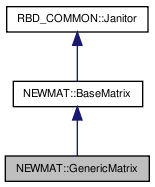# NEWMAT::GenericMatrix Class Reference

`#include <newmat.h>`

Inheritance diagram for NEWMAT::GenericMatrix:[legend]

## Detailed Description

Definition at line 1232 of file newmat.h.

List of all members.

## Public Member Functions

GenericMatrix ()
GenericMatrix (const BaseMatrix &bm)
GenericMatrix (const GenericMatrix &bm)
void operator= (const GenericMatrix &)
void operator= (const BaseMatrix &)
void operator+= (const BaseMatrix &)
void operator-= (const BaseMatrix &)
void operator*= (const BaseMatrix &)
void operator|= (const BaseMatrix &)
void operator&= (const BaseMatrix &)
void operator+= (Real)
GenericMatrixoperator-= (Real r)
void operator*= (Real)
GenericMatrixoperator/= (Real r)
~GenericMatrix ()
void CleanUp ()
void Release ()
GeneralMatrixEvaluate (MatrixType=MatrixTypeUnSp)
MatrixBandWidth BandWidth () const
void swap (GenericMatrix &x)

## Private Member Functions

int search (const BaseMatrix *bm) const

GeneralMatrixgm

class BaseMatrix

## Constructor & Destructor Documentation

 NEWMAT::GenericMatrix::GenericMatrix ( )

Definition at line 1238 of file newmat.h.

 NEWMAT::GenericMatrix::GenericMatrix ( const BaseMatrix & bm )

Definition at line 1239 of file newmat.h.

 NEWMAT::GenericMatrix::GenericMatrix ( const GenericMatrix & bm )

Definition at line 1240 of file newmat.h.

 NEWMAT::GenericMatrix::~GenericMatrix ( )

Definition at line 1252 of file newmat.h.

## Member Function Documentation

 MatrixBandWidth NEWMAT::GenericMatrix::BandWidth ( ) const` [virtual]`

Reimplemented from NEWMAT::BaseMatrix.

Definition at line 457 of file newmat4.cpp.

 void NEWMAT::GenericMatrix::CleanUp ( ) ` [virtual]`

Reimplemented from NEWMAT::BaseMatrix.

Definition at line 1253 of file newmat.h.

 GeneralMatrix * NEWMAT::GenericMatrix::Evaluate ( MatrixType mt = `MatrixTypeUnSp` ) ` [virtual]`

Implements NEWMAT::BaseMatrix.

Definition at line 91 of file newmat5.cpp.

 void NEWMAT::GenericMatrix::operator&= ( const BaseMatrix & X )

Definition at line 615 of file newmat6.cpp.

 void NEWMAT::GenericMatrix::operator*= ( Real r )

Definition at line 643 of file newmat6.cpp.

 void NEWMAT::GenericMatrix::operator*= ( const BaseMatrix & X )

Definition at line 585 of file newmat6.cpp.

Referenced by operator/=().

 void NEWMAT::GenericMatrix::operator+= ( Real r )

Definition at line 630 of file newmat6.cpp.

 void NEWMAT::GenericMatrix::operator+= ( const BaseMatrix & X )

Definition at line 555 of file newmat6.cpp.

Referenced by operator-=().

 GenericMatrix& NEWMAT::GenericMatrix::operator-= ( Real r )

Definition at line 1249 of file newmat.h.

 void NEWMAT::GenericMatrix::operator-= ( const BaseMatrix & X )

Definition at line 570 of file newmat6.cpp.

 GenericMatrix& NEWMAT::GenericMatrix::operator/= ( Real r )

Definition at line 1251 of file newmat.h.

 void NEWMAT::GenericMatrix::operator= ( const BaseMatrix & bmx )

Definition at line 445 of file newmat6.cpp.

 void NEWMAT::GenericMatrix::operator= ( const GenericMatrix & bmx )

Definition at line 438 of file newmat6.cpp.

 void NEWMAT::GenericMatrix::operator|= ( const BaseMatrix & X )

Definition at line 600 of file newmat6.cpp.

 void NEWMAT::GenericMatrix::Release ( )

Definition at line 1254 of file newmat.h.

 int NEWMAT::GenericMatrix::search ( const BaseMatrix * bm ) const` [private, virtual]`

Implements NEWMAT::BaseMatrix.

Definition at line 411 of file newmat4.cpp.

 void NEWMAT::GenericMatrix::swap ( GenericMatrix & x )

Definition at line 1100 of file newmat4.cpp.

Referenced by NEWMAT::swap().

## Friends And Related Function Documentation

 friend class BaseMatrix` [friend]`

Definition at line 1236 of file newmat.h.

## Member Data Documentation

 GeneralMatrix* NEWMAT::GenericMatrix::gm` [private]`

Definition at line 1234 of file newmat.h.

The documentation for this class was generated from the following files:

 newmat11b Generated Mon May 9 04:54:19 2016 by Doxygen 1.6.3